# AQA A Level Maths: Statistics复习笔记3.1.1 Calculating Probabilities & Events

### Probability Basics

#### What do I need to know about probability for AS and A level Mathematics?

• The language used in probability can be confusing so here are some definitions of commonly misunderstood terms
• An experiment is a repeatable activity that has a result that can be observed or recorded; it is what is happening in a question
• An outcome is the result of an experiment
• All possible outcomes can be shown in a sample space – this may be a list or a table and is particularly useful when it is difficult to envisage all possible outcomes in your head

e.g.  The sample space below is for two fairfour-sided spinners whose outcomes are the product of the sides showing when spun.• An event is an outcome or a collection of outcomes; it is what we are interested in happening
• Do note how this could be more than one outcome
e.g. For the spinners above,
the event “the product is -2” has one outcome but
the event “the product is negative” has 6 outcomes

####How do I solve A level probability questions?

•  The big difference with probability at A level is the language and the notation used
•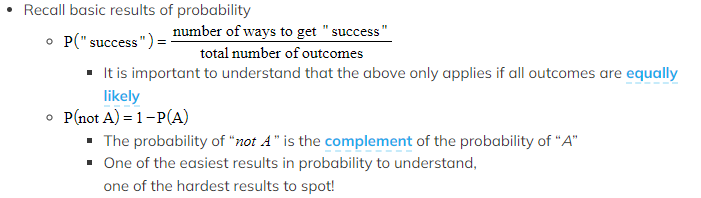Be aware of whether you are using theoreticalprobabilities or probabilities based on the results of several experiments (relativefrequency). You may have to compare the two and make a judgement as to whether there is bias in the experiment.

e.g.        The outcomes from rolling a fair dice have theoretical probabilities but the outcomes from a football match would be based on previous results between the two teams

• Ensure you can interpret common ways of displaying data – from frequency tables, histograms, box plots and other ways to illustrate data
• See Revision Notes       2.1.2 Frequency Tables
2.2.1 Data Presentation
2.2.2 Box Plots & Cumulative Frequency
2.2.3 Histograms
• Be particularly careful when using histograms
• These use frequency density, not frequency
• Using parts of bars may be required due to where class boundaries fall so values will be estimates (using the proportion of the bar needed, sometimes called interpolation)

#### Worked Example

100 skydivers took part in an all-day charity event, with the altitude of the aeroplane at which they jumped from summarised in the histogram below.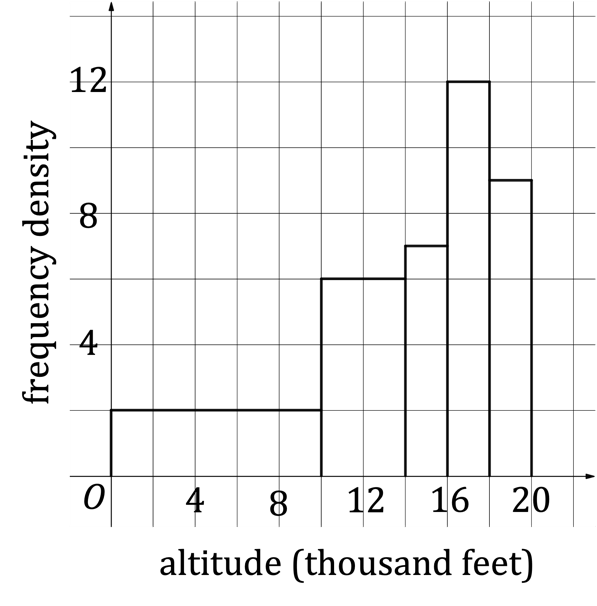(a)Use the histogram to find the probability that a randomly chosen skydiver jumped from the aeroplane at an altitude
(i)between 14 000 and 16 000 feet,
(ii)between 16 000 and 20 000 feet.
(b)Estimate the probability that a randomly chosen skydiver jumped from the aeroplane at an altitude between 13 000 and 15 000 feet.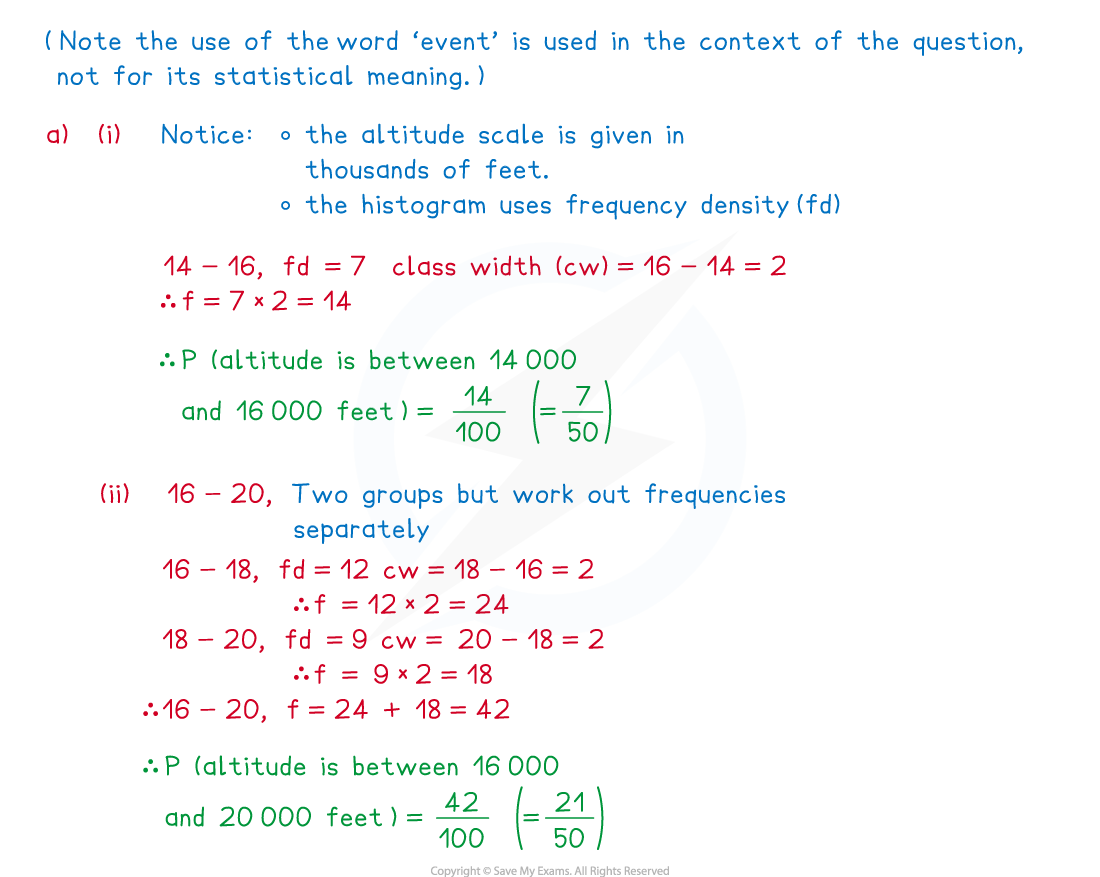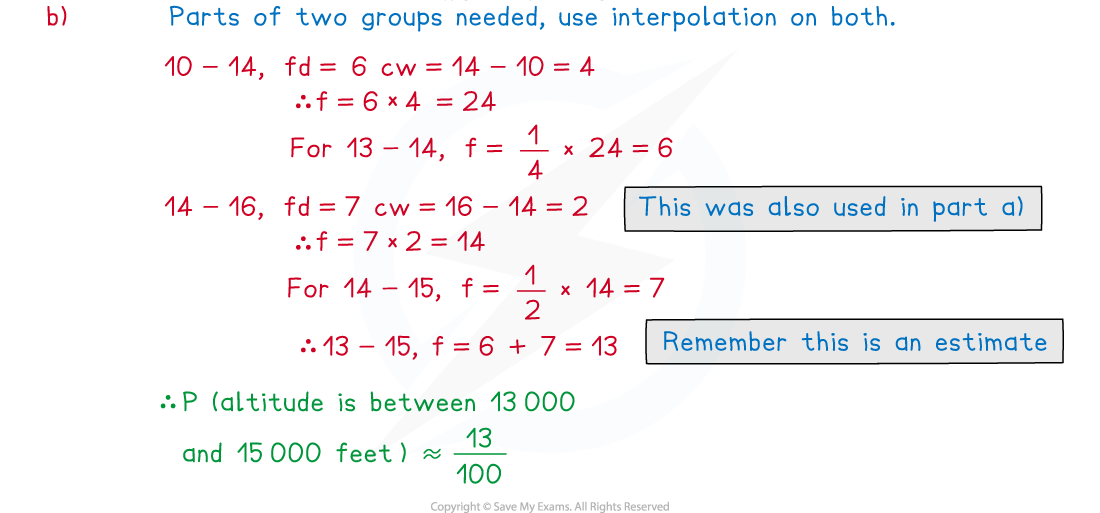#### Exam Tip

• Most probability questions are in context so can be long and wordy; go back and re-read the question, several times, whenever you need to
• Try to get immersed in the context of the question to help understand a problem

### Independent & Mutually Exclusive Events

#### What are independent events?

• Independent eventsdo not affect each other
• For two independent events, the probability of one event happening is unaffected by the outcome of the other event

e.g.    The events “rolling a 6 on a dice” and “flipping heads on a coin” are    independent - the outcome “rolling a 6” does not affect the probability of the outcome “heads” (and vice versa)

• For two independent events, A and B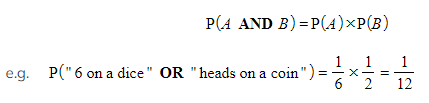• Independent events could refer to events from different experiments

####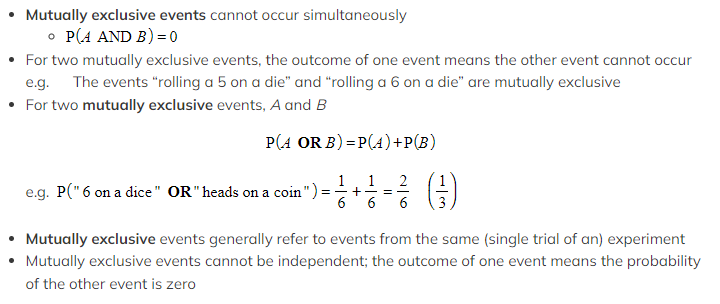How do I solve problems involving independent and mutually exclusive events?

• Make sure you know the statistical terms – independent and mutually exclusive
• Remember
•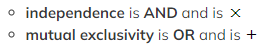Solving problems will require interpreting the information given and the application of the appropriate formula
• Information may be explained in words or by diagram(s)

(including Venn diagrams – see Revision Note 3.1.2 Venn Diagrams)

• Showing or determining whether two events are independent or mutually exclusive are also common
• To do this you would show the relevant formula is true

Just for fun …

• A well-known sports TV broadcaster used to advertise their football matches as either “Live and exclusive” or “Exclusively live” – can you tell the difference?
• “Live and exclusive” meant that the broadcaster was airing the football match live and was the only broadcaster allowed to air any of the match at any time.
“Exclusively live” meant that the broadcaster was the only one airing the football match live, but other broadcasters would be able to air any of the match afterwards.

#### Worked Example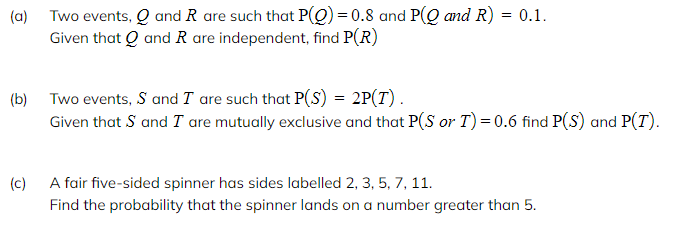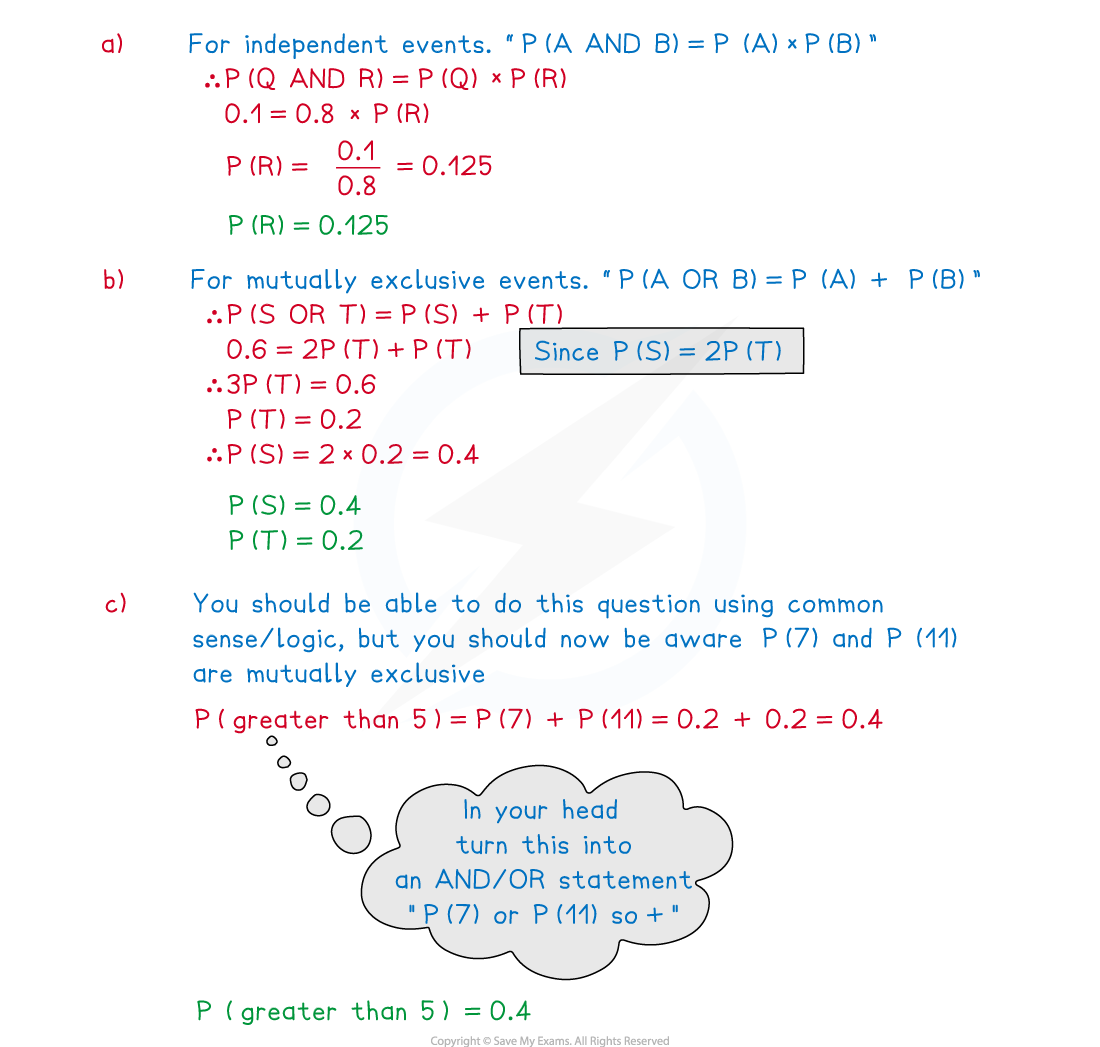#### Exam Tip

• Try to rephrase questions in your head in terms of AND and/or OR !
e.g.      A fair six-sided die is rolled and a fair coin is flipped.
“Find the probability of obtaining a prime number with heads.”

would be

“Find the probability of rolling a 2 OR a 3 OR a 5 AND heads.”